# J(32,2,4) Generalized Johnson Graph

The Second DIMACS Implementation Challenge: 1992-1993

Originator: Panos Pardalos

NP Hard Problems: Maximum Clique, Graph Coloring, and Satisfiability, The Second DIMACS Implementation Challenge: 1992-1993.

A Johnson graph with parameters n, w, d has a node for every binary vector of length n with exactly w 1s. Two vertices are adjacent if and only if their hamming distance is at least d.

(70 vertices, 1855 edges)

## Examples

### Basic Examples

Retrieve the graph:

 In:=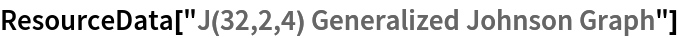Out=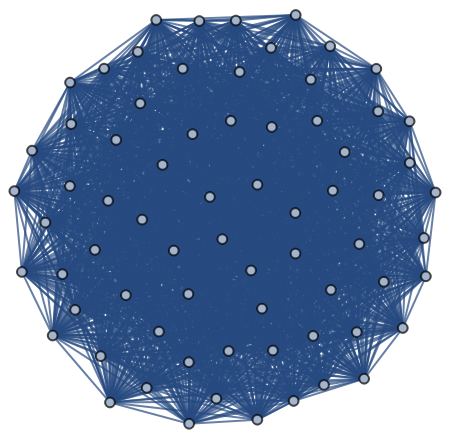Summary properties:

 In:=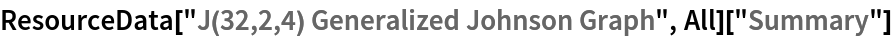Out=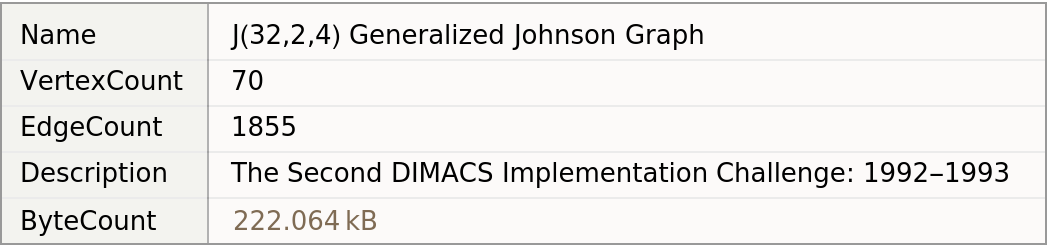### Basic Applications

Find the maximum clique:

 In:=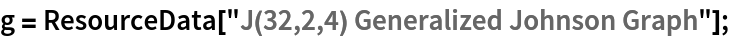In:=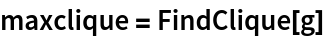Out=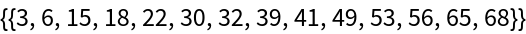Show the maximum clique:

 In:=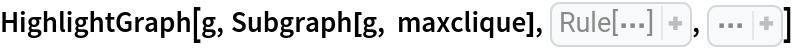Out=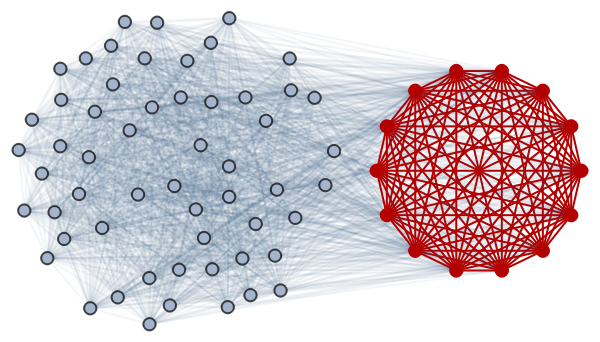Wolfram Research, "J(32,2,4) Generalized Johnson Graph" from the Wolfram Data Repository (2019)